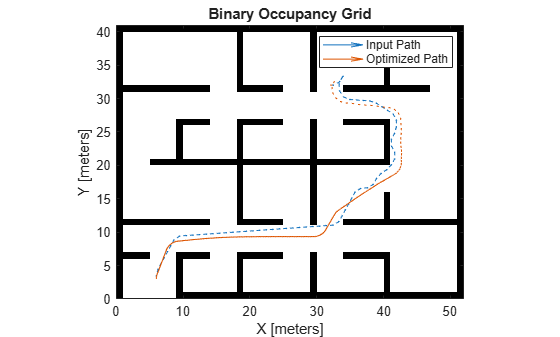# optimizePath

Optimize path while maintaining safe distance from obstacle

## Syntax

``optPath = optimizePath(path)``
``optPath = optimizePath(path,map)``
``optPath = optimizePath(path,options)``
``optPath = optimizePath(path,map,options)``
``[optPath,kineticInfo] = optimizePath(___)``
``[optPath,kineticInfo,solutionInfo] = optimizePath(___)``

## Description

````optPath = optimizePath(path)` optimizes the travel time and smoothness of the specified `path` for car-like robots in an empty map for the default parameters. The number of states in the `optPath` can be different than in the `path`.```
````optPath = optimizePath(path,map)` optimizes the `path` in the specified `map` for the default parameters. The returned optimized path `optPath` tries to maintain a safety margin to obstacles in the map `map`.```
````optPath = optimizePath(path,options)` optimizes the `path` in an empty map by parameters specified in `options`. Parameters related to trajectory, obstacle, and solver, along with the weights of those parameters can be specified in `options` as an `optimizePathOptions` object.```

example

````optPath = optimizePath(path,map,options)` optimizes the `path` in the specified `map` for by parameters specified in `options`.```
````[optPath,kineticInfo] = optimizePath(___)` also returns `kineticInfo` as a structure that contains the kinetic information of the optimized path.```
````[optPath,kineticInfo,solutionInfo] = optimizePath(___)` also returns `solutionInfo` as a structure that contains the solution information of the optimized path.```

## Examples

collapse all

Setup Environment

Load a map into the workspace.

`map = load("exampleMaps.mat").complexMap;`

Create a binary occupancy map.

`map = binaryOccupancyMap(map);`

Create a state validator object.

`stateValidator = validatorOccupancyMap;`

Assign the map to the state validator object.

`stateValidator.Map = map;`

Set the validation distance for the validator.

`stateValidator.ValidationDistance = 0.01;`

Plan Path

Initialize the `plannerHybridAStar` object with the state validator object. Specify the `MinTurningRadius` property of the planner as 2 meters.

`planner = plannerHybridAStar(stateValidator,MinTurningRadius=2);`

Define start and goal poses as [x y theta] vectors. x and y specify the position in meters, and theta specifies the orientation angle in radians.

```start = [6 3 pi/2]; goal = [32 32 0];```

Plan a path from the start pose to the goal pose.

```path = plan(planner,start,goal); inpath = path.States;```

Optimize Path

Configure options for optimization.

`options = optimizePathOptions`
```options = optimizePathOptions Trajectory Parameters MaxPathStates: 200 ReferenceDeltaTime: 0.3000 MinTurningRadius: 1 MaxVelocity: 0.4000 MaxAngularVelocity: 0.3000 MaxAcceleration: 0.5000 MaxAngularAcceleration: 0.5000 Obstacle Parameters ObstacleSafetyMargin: 0.5000 ObstacleCutOffDistance: 2.5000 ObstacleInclusionDistance: 0.7500 Solver Parameters NumIteration: 4 MaxSolverIteration: 15 Weights WeightTime: 10 WeightSmoothness: 1000 WeightMinTurningRadius: 10 WeightVelocity: 100 WeightAngularVelocity: 10 WeightAcceleration: 10 WeightAngularAcceleration: 10 WeightObstacles: 50 ```

Set the minimum turning radius value as same as in the planner.

`options.MinTurningRadius = 2;`

Specify the maximum number of poses allowed in the optimized path.

`options.MaxPathStates = size(inpath,1) * 3;`

Maintain a safe distance of `0.75` meters from the obstacles.

`options.ObstacleSafetyMargin = 0.75;`

Optimize the path generated by the planner.

`optpath = optimizePath(inpath,map,options);`

Visualize

Visualize input path and optimized path in the map.

```show(map) hold on quiver(inpath(:,1),inpath(:,2),cos(inpath(:,3)),sin(inpath(:,3)),0.1); quiver(optpath(:,1),optpath(:,2),cos(optpath(:,3)),sin(optpath(:,3)),0.1); legend("Input Path","Optimized Path")```## Input Arguments

collapse all

Path states, specified as a real-valued M-by-2 matrix of the form [x y] or M-by-3 matrix of the form [x y theta]. x and y specify the position in meters. theta specifies the orientation in radians. M is the number of states in the path. The number of states in the path must be greater than or equal to 3.

Data Types: `single` | `double`

Map representation, specified as a `binaryOccupancyMap`, `occupancyMap`, or `vehicleCostmap` (Automated Driving Toolbox) object. This object represents the environment of the vehicle.

Optimization options, specified as `optimizePathOptions` object.

## Output Arguments

collapse all

Path states, returned as a real-valued N-by-2 matrix of the form [x y] or N-by-3 matrix of the form [x y theta]. x and y specify the position in meters. theta specifies the orientation in radians. N is the number of states in the path. The number of states in the `optPath` can be different than in the `path`.

Kinetic Information, returned as a structure. The fields of the structure are:

Fields of `kineticInfo`

FieldsDescription
`TimeStamps`

Time corresponding to the pose in the optimized path.

`Velocity`

Velocity to travel between two consecutive poses.

`AngularVelocity`

Angular Velocity between two consecutive poses.

Data Types: `struct`

Solution Information, returned as a structure. The fields of the structure are:

Fields of `solutionInfo`

FieldsDescription
`NumIterations`

Number of iterations performed.

`Cost`

Final cost of the path.

Data Types: `struct`

## Version History

Introduced in R2022a